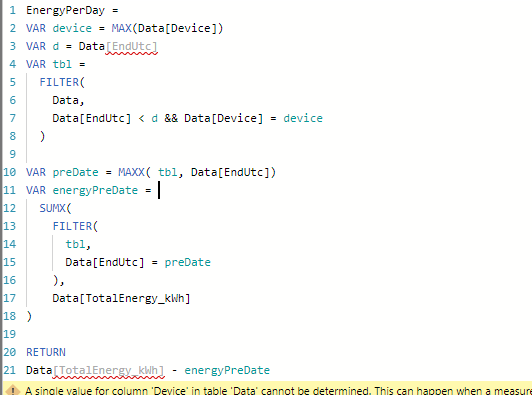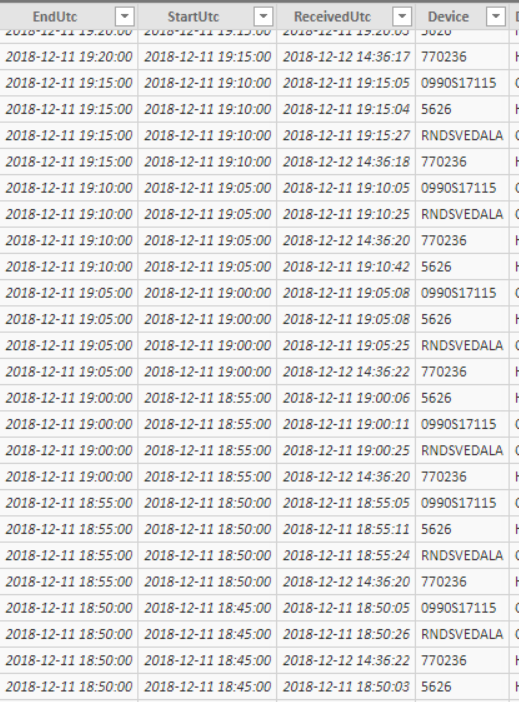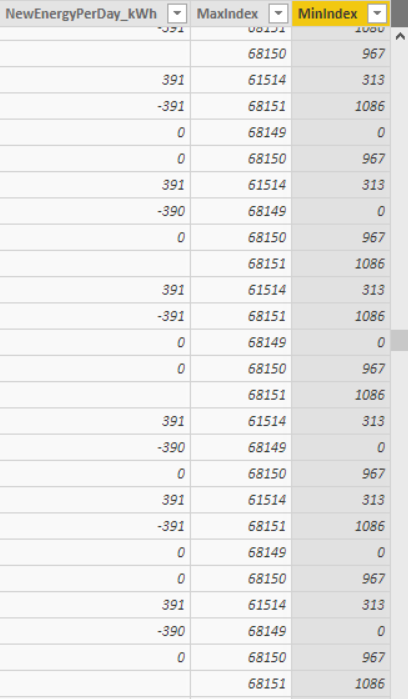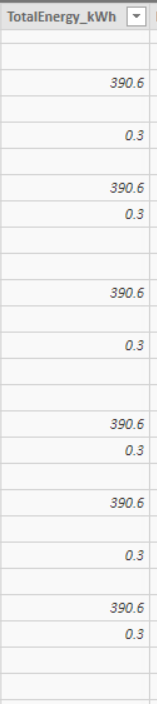cancel
Showing results for
Did you mean:

Fabric is Generally Available. Browse Fabric Presentations. Work towards your Fabric certification with the Cloud Skills Challenge.Anonymous
Not applicable

## Measure to calculate current value minus previous row for a specific device

Hey!

I have the same problem for some time now that I really can't solve. I am doing a calculation to calculate the total energy,

I have following as example:

I have a column with an accumulative TotalEnergy for every device, I have the devices as dirfferent name, Index and I made a Rank with following formula (Where EndUtc is Date and time)

Rank = RANKX(FILTER(Data, Data[Device] = EARLIER(Data[Device])), Data[EndUtc],,ASC)

I wanna make a calculation NEWENERGYPERDAY: as for device A, 365-364 = 1, 364-364=0 etc, for every row.I have tried many different calculations, as for example:

NewEnergyPer300s_kWh =
VAR minIndx = CALCULATE (
MAX ( Data[Index] ),
ALLEXCEPT ( Data, Data[Device] ),
Data[Index] < EARLIER (Data[Index] )
)

RETURN

IF (
ISBLANK ( minIndx ),
BLANK (),
Data[TotalEnergy_kWh]
- CALCULATE (
SUM ( Data[TotalEnergy_kWh] ),
Data[Index] = minIndx,
ALL ( Data )
)
)

which worked fine, except that I am getting not enough memory capacity, which is weird cause I don't even have too much data, and when I find other formulas and using function EARLIER I get the same issue evrytime, is it possible to write this formula or similar as a measure and not a column?

And also if there are any better way to write the calculation that will be better for the memory capcity?

Thanks! Feeling a bit lost with this issue.

Kind regards

2 ACCEPTED SOLUTIONSResponsive Resident

Hi again,

Can you share your file? I am sorry but I think that I have overlooked some important facts in original post, but if you have created a Index column that increases with every new entry per device you should be able to use this calculated column. And perhaps you do not have to use the device varible at all (i.e. if i Index contains unique values)

```EnergyPerDay =
VAR device = Data[Device]    // could be skipped
VAR ind = Data[Index]
VAR preInd= ind-1
VAR energyPreDate  =
SUMX(
FILTER(
Data,
Data[Index] = preInd       && Data[Device] = device   // could also be skipped
),
Data[TotalEnergy_kWh]
)

RETURN
Data[TotalEnergy_kWh] - energyPreDate```

Best regars,

KristjanMicrosoft

Hi @Anonymous

You may try to create below measures:

```NextValue =
CALCULATE (
SUM ( Data[TotalEnergy_kWh] ),
FILTER ( ALLEXCEPT ( Data, Data[Device] ), Data[Rank] = MAX ( Data[Rank] ) - 1 )
)
```
```NEWENERGYPERDAY =
IF (
ISBLANK ( [NextValue] ),
BLANK (),
SUM ( Data[TotalEnergy_kWh] ) - [NextValue]
)
```Regards,

Cherie

Community Support Team _ Cherie Chen
If this post helps, then please consider Accept it as the solution to help the other members find it more quickly.
12 REPLIES 12Microsoft

Hi @Anonymous

You may try to create below measures:

```NextValue =
CALCULATE (
SUM ( Data[TotalEnergy_kWh] ),
FILTER ( ALLEXCEPT ( Data, Data[Device] ), Data[Rank] = MAX ( Data[Rank] ) - 1 )
)
```
```NEWENERGYPERDAY =
IF (
ISBLANK ( [NextValue] ),
BLANK (),
SUM ( Data[TotalEnergy_kWh] ) - [NextValue]
)
```Regards,

Cherie

Community Support Team _ Cherie Chen
If this post helps, then please consider Accept it as the solution to help the other members find it more quickly.Responsive Resident

Hi agnes_,

You can try this,

```EnergyPerDay =
VAR device = Data[Device]
VAR d = Data[Date]
VAR tbl =
FILTER(
Data,
Data[Date] < d && Data[Device] = device
)

VAR preDate = MAXX( tbl, Data[Date])
VAR energyPreDate =
SUMX(
FILTER(
tbl,
Data[Date] = preDate
),
Data[TotalEnergy_kWh]
)

RETURN
Data[TotalEnergy_kWh] - energyPreDate

```

If this works please LIKE and mark as solutions.

P.s. if there is no gap in the dates, you can change VAR preDate = MAXX( tbl, Data[Date]) to preDate = d-1 and then use preDate in the tbl varible right away.

```EnergyPerDay =
VAR device = Data[Device]
VAR d = Data[Date]
VAR preDate = d-1
VAR tbl =
FILTER(
Data,
Data[Date] = preDate && Data[Device] = device
)

VAR energyPreDate =
SUMX(
tbl,
Data[TotalEnergy_kWh]
)

RETURN
Data[TotalEnergy_kWh] - energyPreDate```

Regards,

KristjanAnonymous
Not applicable

Thanks!

But everytime I try this as a measure I get the same problem, that PowerBI can't find  the column tables, and the names are correct: It find it at some places and some not.

If I put max, it can find it though:Anonymous
Not applicableAnd as a new column, same here not enough memory to complete the operation, which is weird cause I have other files that have more data then this one.Anonymous
Not applicable
Hello my question won't help but im curious : the same formula works on a bigger table than the one with memory error ? thank youAnonymous
Not applicable

Yes, the other table uses this formula for the same type of calculation (someone else have made it though), but here are only one device!

```Consumed Energy =
VAR
//Our Base Energy Consumption Value
BaseValue = xlsx[ Stat_EnergyConsumptionSinceMantleChange]
VAR
//The date our BaseValue is based upon
BaseDate=xlsx[DateTime]
VAR
//The date of the value we're planning on subtracting from our base value
DateCheck=LOOKUPVALUE(xlsx[DateTime],xlsx[Index],xlsx[Index]-1)
VAR
ValueToSubtract = IF(
'xlsx'[Index]=0,xlsx[ Stat_EnergyConsumptionSinceMantleChange], //If index = 0 we're at the beginning of our list
LOOKUPVALUE(
xlsx[ Stat_EnergyConsumptionSinceMantleChange],
xlsx[Index],
xlsx[Index]-1)
)

RETURN
IF(FORMAT(BaseDate,"YYYY-MM-DD")=FORMAT(DateCheck,"YYYY-MM-DD"), //If we're comparing values of the same date
IF(ISBLANK(ValueToSubtract), //If value we're trying to subtract is blank
BaseValue-BaseValue, // return basevalue - basevalue
IF(ValueToSubtract>BaseValue, //If value we're trying to subtract is larger then our base value
BaseValue-BaseValue, //return basevalue - basevalue
BaseValue-ValueToSubtract) //Otherwise return the difference
),
BaseValue-BaseValue) //If the dates are NOT the same - return 0

```Responsive Resident

Hi again,

Can you share your file? I am sorry but I think that I have overlooked some important facts in original post, but if you have created a Index column that increases with every new entry per device you should be able to use this calculated column. And perhaps you do not have to use the device varible at all (i.e. if i Index contains unique values)

```EnergyPerDay =
VAR device = Data[Device]    // could be skipped
VAR ind = Data[Index]
VAR preInd= ind-1
VAR energyPreDate  =
SUMX(
FILTER(
Data,
Data[Index] = preInd       && Data[Device] = device   // could also be skipped
),
Data[TotalEnergy_kWh]
)

RETURN
Data[TotalEnergy_kWh] - energyPreDate```

Best regars,

KristjanResponsive Resident

Hi again,

@Anonymous I am sorry but I do not understand what you mean.

@Anonymous, please let me know if the second calculated column worked.

Regards,

KristjanAnonymous
Not applicable

Yes, second formula worked without getting the memory capacity, but the results gets very high negative values, and the values should not be high at all, and positive, maybee I should use some LOOKUPVALUE, instead of the SUMX one?Anonymous
Not applicable
My question wasn't for you 🙂
Agnes said that she got an memory error with the formula. But she had bigger tables. I wanres to be sure that she did run the actual formula on the big table as well.

If not clear, forget it, not a big deal ! 😉Responsive Resident

Hi again,

If Data[EndUtc] only contains one row for each device and there are no missing dates you should try the second version of the calculated column. How many rows do you have in your table?

Regards,

kristjanAnonymous
Not applicable

[EndUtc] have have data every 300s (every 5th minute of the day) but only one EndUtc for every device, so if there are five devices, there are five 2019-07-30 13:25:00 stamps for example.

I have 68,152 rows, 30 Columns, The memory capacity error occurs with Earlier function.

Another PowerBI I have been working with the same type of calculation

```NewEnergyPerDay_kWh =

var maxIndex =CALCULATE( MIN('Data'[MinIndex]),
FILTER('Data','Data'[MinIndex]=EARLIER('Data'[MinIndex])))
VAR minVal='Data'[TotalEnergy_kWh]
var maxVal= IF('Data'[Index]=maxIndex,
LOOKUPVALUE('Data'[TotalEnergy_kWh],'Data'[Index],'Data'[Index]),
LOOKUPVALUE('Data'[TotalEnergy_kWh],'Data'[Index],'Data'[Index]+1))
RETURN
CALCULATE(DIVIDE(minVal-maxVal,1,0),FILTER('Data','Data'[MinIndex]=EARLIER('Data'[MinIndex])), ALLEXCEPT(Data,Data[Device]))```

where:

```MaxIndex = CALCULATE(MAX('Data'[Index]),ALLEXCEPT('Data','Data'[Device]))

MinIndex = CALCULATE(MIN('Data'[Index]),ALLEXCEPT('Data','Data'[Device]))```

there I don't get any memory error, but still some error:Announcements#### Power BI Monthly Update - November 2023

Check out the November 2023 Power BI update to learn about new features.#### Fabric Community News unified experience

Read the latest Fabric Community announcements, including updates on Power BI, Synapse, Data Factory and Data Activator.#### The largest Power BI and Fabric virtual conference

130+ sessions, 130+ speakers, Product managers, MVPs, and experts. All about Power BI and Fabric. Attend online or watch the recordings.Top Solution Authors
Top Kudoed Authors
Users online (1,707)